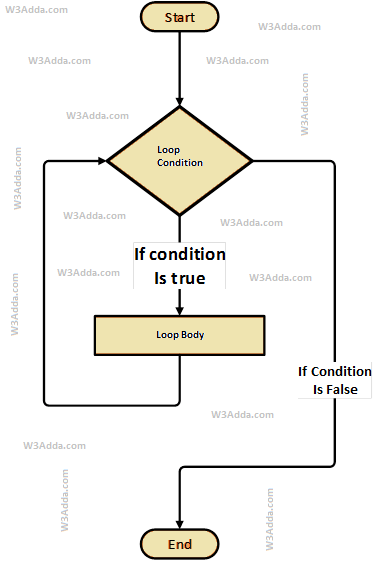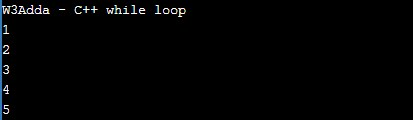# C++ while Loop

In this tutorial you will learn about the C++ while Loop and its application with practical example.

## C++ While Loop

The while loop will execute a block of statement as long as a test expression is true. While loop is useful when the number of iterations can not be predicted beforehand. The while loop evaluates test expression at beginning of each pass.

## C++ While Loop Flow DiagramSyntax:-

Here, Condition is a Boolean expression that results in either True or False, if it results in True then statements inside loop body are executed and condition is evaluated again. This process is repeated until the condition is evaluated to False. If the condition results in False then execution is skipped from loop body and while loop is terminated.

Example:-

Here, we have initialized ctr and maxCtr to 1 and 5 respectively, in the next statement we have while loop, that checks the condition ctr <= maxCtr for each of the iteration. If the test condition returns True, the statements inside loop body are executed and if test condition returns False then the while loop is terminated. Inside the loop body we have incremented the ctr value by one for each if the iteration. When we run the above c++ program, we see the following output –

Output:-In this tutorial we have learn about the C++ while Loop and its application with practical example. I hope you will like this tutorial.